5.5.6. Chemically inhomogeneous equilibrium models

The heavier an ion is, the slower its thermal motion in the intracluster gas will be. As a result, heavy ions will tend to drift toward the center of the cluster. In Section 5.4.5 the drift rate was calculated and shown to be rather slow, although the precise value depends on cluster parameters. Further, the intracluster magnetic field may be very effective in retarding any sedimentation of heavy elements. Nonetheless, it is possible that heavier elements may have drifted to the cluster center in some cases, or been introduced into the intracluster gas quite near the center. Eventually, the motion of heavy ions towards the cluster center will stop when the resulting charge separation produces an electric field that cancels the effect of the gravitational field.

Abramopoulos et al. (1981) have calculated chemically inhomogeneous models for the intracluster gas in thermodynamic equilibrium. The temperature of the gas is therefore constant, both spatially and among the various ions and electrons. These models satisfy the hydrostatic equation (5.55) for the total pressure, but this does not uniquely define the chemically inhomogeneous model, since any value of the total pressure can be attributed to an infinite number of different combinations of partial pressures of different ions. In thermodynamic equilibrium, the number density of any ion is given by the Boltzmann equation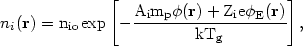(5.86)

where Ai, zi, and nio are the mass, charge, and central density of the ion i. The cluster gravitational potential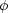and electrical potentiale are defined in this equation to be zero at the cluster center. The gravitational potential is assumed to be fixed and known, as in most of the hydrostatic models discussed in the previous sections. The electrical potential is given as a solution of Poisson's equation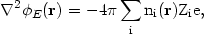(5.87)

where the sum includes electrons as one of the ionic species. Because the coupling constant for electrical forces exceeds that of gravitational forces by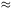1040, while the fraction of the cluster mass due to intracluster gas is much greater than the inverse of this, the electrical potential will generally be quite smallE-mp / e, and a sufficient approximation to the solution of equation (5.87) is to assume that the gas is very nearly electrically neutral: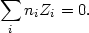(5.88)

The electric potential is then given by requiring a simultaneous solution of equations (5.86) and (5.88), and requiring that the total abundanceni dV of each of the ions be fixed.

In the case where the plasma can be assumed to consist only of protons and electrons, with the abundances of all heavy elements being so low that they do not contribute to the potential, the solution for the ion and electron densities is the same as the isothermal hydrostatic solution given above (equation 5.63), the electric potential ise = -mp/ 2e, and the densities of any trace heavy elements will be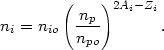(5.89)

Unfortunately, the abundance of helium certainly can not be treated as insignificant, and other heavier elements may contribute to the electric potential near the cluster core. Equations (5.86) and (5.88) have been solved numerically by Abramopoulos et al. (1981), assuming an analytic King potential for the cluster, taking the gas temperature to be= 2/3 (equation 5.64), and assuming a range of heavy element abundances from pure hydrogen and helium to solar. They find that the heavy elements are strongly concentrated to the cluster core. To balance the resulting increase in the charge density, the electrons also must be centrally condensed.

The concentration of heavy elements to the cluster core, where the electron density is high, results in an increase in the X-ray line intensity for a given set of heavy element abundances. As a result, Abramopoulos et al. (1981) argue that the observed line strengths could be produced by gas with iron abundances of only 1/20 of solar.